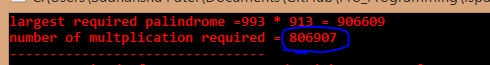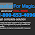## Friday, 27 March 2015

### Find the largest palindrome made from the product of two 3-digit numbers.

This Is  very easy ProjectEuler Problem. but it worth trying. First of all Try it by yourself then back to us even if you succeed because getting answer should not be our only objective. How efficient is our approach also matters.

First Approach People try is find largest palindrome in range 100*100 to 999*999 this is a very obvious but wrong approach because all numbers in this range are not multiple of 2 3 digit number .

Try this Python Code to check it out

1. #Python 2.7
2. #beginner to computer Science
3. limit=999*999
4. base=100
5. def ispalindrom(n):
6.     st=str(n)
7.     if(st==st[::-1]):
8.         return True
9.     else:
10.         return False
11. for i in range(limit,base,-1):
12.     if ispalindrom(i):
13.         print i
14.         break
\
Now try Another approach :> Check all number that is multiple of  two 3 digit number. and find largest palindrome number among them.
It will give correct result but at what cost
We know multiplication is very costly in computation & this approach takes about =806907  multiplication of two three digit number
Check it out by yourself

1. //C-Program
2. //Very costly with respect to calculation required
3. #include<stdio.h>
4. #include<string.h>
5. int isPalindrome_num(long long int n){
6.         /*
7.           ****<string.h>
8.            Used to check number is palindrome or not
9.            """
10.              isPalindrome_num(n)
11.              return 1 if palindrome
12.              return 0 if not palindrome
13.            """
14.         */
15.         char a;
16.         char b;
17.         sprintf(a,"%d",n); //convert number in string & store it in array a
18.         strcpy(b,a); //copy b<-a
19.         _strrev(b);  //reverse b
20.         if(strcmp(a,b)==0) //compare
21.             return 1;
22.         else
23.             return 0;
24. }

25. int main(){
26.         int i,j,p,count=0,t1,t2;
27.         int max=10001; //least 4 digit number
28.         for(i=999;i>100;i--){
29.                 for(j=999;j>100;j--){
30.                         p=i*j;
31.                         count++;
32.                         //printf("%d, ",p);
33.                         if(p>max && isPalindrome_num(p)==1){
34.                                 max=p;
35.                                 t1=i;
36.                                 t2=j;
37.                                 break;
38.                         }
39.                 }
40.         }
41.         printf("largest required palindrome =%d * %d = %d",t1,t2,max);
42.         printf("\nnumber of multplication required = %d",count);
43.         return 0;
44. }YOu Can See number of multiplication required
But We can Very effectively reduced number of multiplication required by eliminatic case.we don't need to check .
let we find an palindrome X then reduce all possible multiplication which produce result less than
Then you can see how efficient your code become .
Check it Out.

1. //C-Program
2. //Optimize number of multiplication required
3. #include<stdio.h>
4. #include<string.h>
5. int isPalindrome_num(long long int n){
6.         /*
7.           ****<string.h>
8.            Used to check number is palindrome or not
9.            """
10.              isPalindrome_num(n)
11.              return 1 if palindrome
12.              return 0 if not palindrome
13.            """
14.         */
15.         char a;
16.         char b;
17.         sprintf(a,"%d",n); //convert number in string & store it in array a
18.         strcpy(b,a); //copy b<-a
19.         _strrev(b);  //reverse b
20.         if(strcmp(a,b)==0) //compare
21.             return 1;
22.         else
23.             return 0;
24. }
25. int main(){
26.         int i,j,p,count=0,t1,t2;
27.         int max=10001; //least 4 digit number
28.         for(i=999;i>100;i--){
29.                 for(j=999;j>100;j--){
30.                         p=i*j;
31.                         count++;
32.                         if(p<max) //stop mult when p<max it is worthless as we want largest
33.                             break;
34.                         else
35.                             if(isPalindrome_num(p)==1){ //if p>max & it is a palindrom
36.                                     max=p; //update max
37.                                     t1=i;  //just for printing result
38.                                     t2=j;
39.                                     break;
40.                                }
41.                 }
42.         }
43.         printf("largest required palindrome =%d * %d = %d",t1,t2,max);
44.         printf("\nnumber of multplication required = %d",count);
45.         return 0;
46. }Optimize code >largest palindrome
You can see slight Modification reduce number of multiplication by about 88 times :)

Please Suggest If you found Any Bug or you know better approach to solve it .
Thank You

1.This comment has been removed by a blog administrator.

2.This comment has been removed by a blog administrator.

3.This comment has been removed by a blog administrator.

4.Magicjack Support 1-800-653-4096,
Customer Support For Magicjack
COMPLETEPCSOLUTION is one of the best way to resolve
all problems like magicjack technical support,s
magicjack customer suppport and installation,
magicjack contact number

THANKS FOR UR GREAT COMMENT

Blogger Widgets